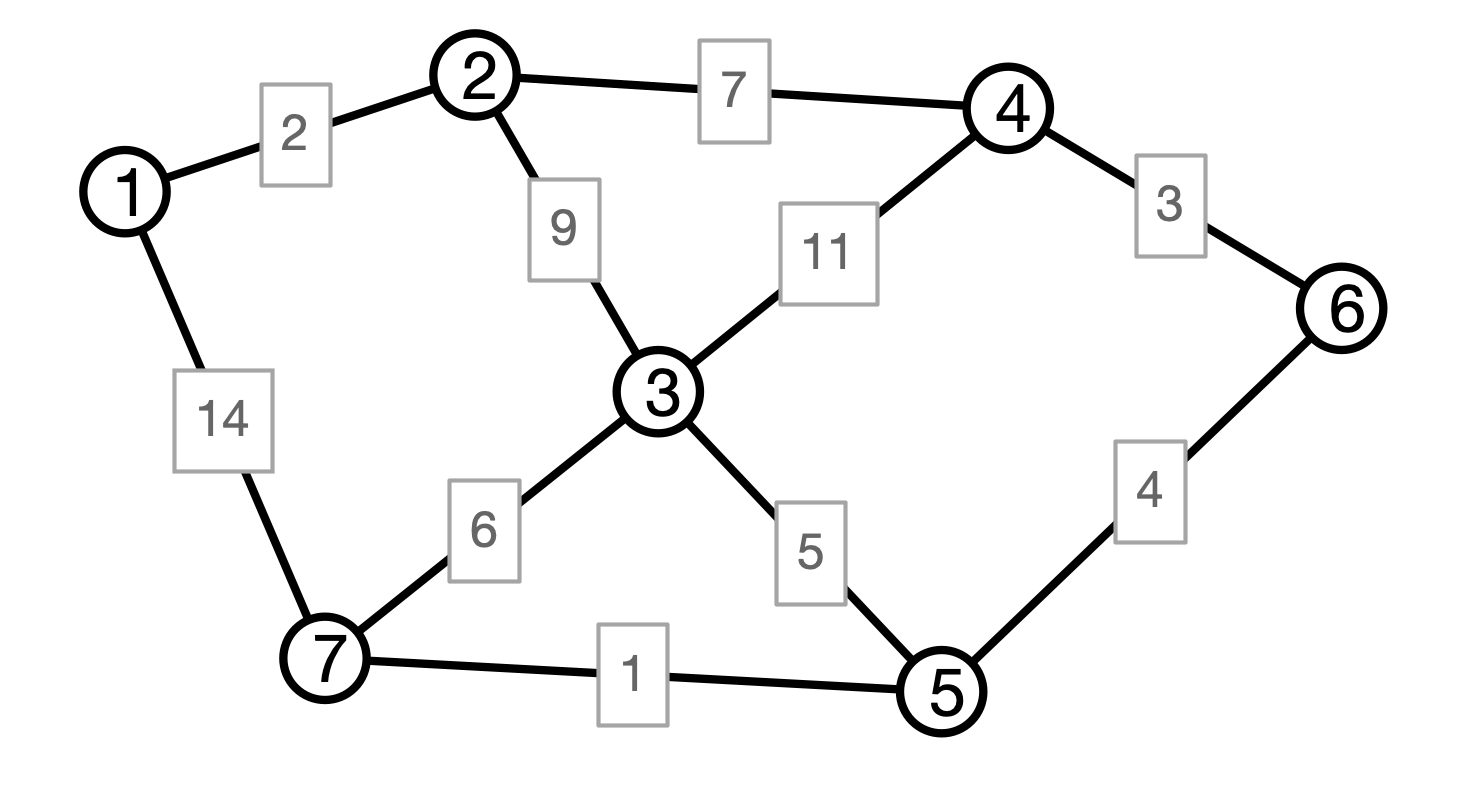# Lecture 20: Minimum Spanning Trees, Part 3

### COSC 311 Algorithms, Fall 2022

$\def\compare{ {\mathrm{compare}} } \def\swap{ {\mathrm{swap}} } \def\sort{ {\mathrm{sort}} } \def\insert{ {\mathrm{insert}} } \def\true{ {\mathrm{true}} } \def\false{ {\mathrm{false}} } \def\BubbleSort{ {\mathrm{BubbleSort}} } \def\SelectionSort{ {\mathrm{SelectionSort}} } \def\Merge{ {\mathrm{Merge}} } \def\MergeSort{ {\mathrm{MergeSort}} } \def\QuickSort{ {\mathrm{QuickSort}} } \def\Split{ {\mathrm{Split}} } \def\Multiply{ {\mathrm{Multiply}} } \def\Add{ {\mathrm{Add}} } \def\cur{ {\mathrm{cur}} } \def\gets{ {\leftarrow} }$

## Announcements

1. Masks still required in class
2. No class on Monday 10/24
3. HW 03, Question 1:
• $n = 2^B$
• array contains $0, 1, \ldots, n - 1$ (not in order)
• values represented as $B$ bit numbers
4. HW 03 now due Sunday

## Last Time

Prim’s algorithm for Minimum Spanning Trees:

• Grow a tree from an arbitrary seed vertex
• Each step, add minimum weight edge out of tree

Cut Claim:

• if $T$ an MST, $U, V - U$ a cut, $e$ min weight cut edge
• then $T$ contains $e$

Prim correctness follows from cut claim

## MSTs, Another Way

Prim:

• Grow tree greedily from a single seed vertex
• Maintain a (connected) tree

Edge Centric View:

• Maintain a collection of edges (not necessarily a tree)
• Add edges to collection to eventually build an MST

Questions:

• How to prioritize edges?
• How to determine whether or not to include an edge?

## Picture## Kruskal’s Algorithm

  Kruskal(V, E, w):
C <- collection of components
initially, each vertex is own component
F <- empty collection
# iterate in order of increasing weight
for each edge e = (u, v) in E
if u and v are in different components then
merge components containing u and v
endif
endfor
return F


## Kruskal Illustration## Kruskal Correctness I

Claim 1. Every edge added by Kruskal must be in every MST.

Why?

• Suppose $e = (u, v)$ added by Kruskal
• Consider the cut $U, V - U$ where $U$ is $u$’s component
• $e$ is lightest edge across the cut (why?)
• therefore $e$ must be in MST (why?)

## Kruskal Correctness II

Claim 2. Kruskal produces a spanning tree.

Why?

• edges added by Kruskal do not contain cycles (why?)

• edges added by Kruskal connect graph (why?)

## Conclusion

Theorem. Kruskal’s algorithm produces an MST.

Next Question. How could we implement Kruskal’s algorithm efficiently? What is its running time?

## Kruskal’s Algorithm

  Kruskal(V, E, w):
C <- collection of components
initially, each vertex is own component
F <- empty collection
# iterate in order of increasing weight
for each edge e = (u, v) in E
if u and v are in different components then
merge components containing u and v
endif
endfor
return F


## Costly Operations

1. Get edges in order of increasing weight
2. Determine if $u$ and $v$ are in same component
3. Merge two components

Question. How to get edges in order of increasing weight?

## Maintaining Components

Idea. For each component, designate a leader

• leader is a vertex in its component
• maintain an array that stores each vertex’s component’s leader
• leader[i] = v means that v is leader of i’s component

Question. How to check if vertices i and j are in the same component? Running time?

## Merging Components

Question. How to merge two components?

## Merging Components Efficiently?

For each leader, maintain list of vertices in its component.

To merge components with leaders $u$ and $v$:

1. choose $u$ or $v$ to be leader of merged component
• how?
2. if $u$ is new leader
• for each vertex $x$ on $v$’s list
• add $x$ to $u$’s list
• set $x$’s leader to $v$

Question. Running time?

## Merging Strategy

When merging components with leaders $u$ and $v$, new leader is leader of larger component

Claim. If $x$ is relabeled $k$ times, then $x$’s component has size at least $2^k$.

## Consequence

Claim. If $x$ is relabeled $k$ times, then $x$’s component has size at least $2^k$.

Consequence 1. If $x$’s component has size $\ell$, then $x$ was relabeled at most $\log \ell$ times.

Consequence 2. Running time of all merge operations in Kruskal is $O(n \log n)$

## Conclusion

Theorem. Kruskal’s algorithm can be implemented to run in time $O(m \log n)$ in graphs with $n$ vertices and $m$ edges.

Remark. More efficient data structures for merging sets exist

• “Union-find” ADT, “disjoint-set forest” data structure
• time to perform merges is $O(n \alpha(n))$
• $\alpha(n)$ is “inverse Ackerman function”
• $\alpha(n)$ grows so slowly, it is practically constant

## Next Time

• Interval Scheduling (recorded lecture)